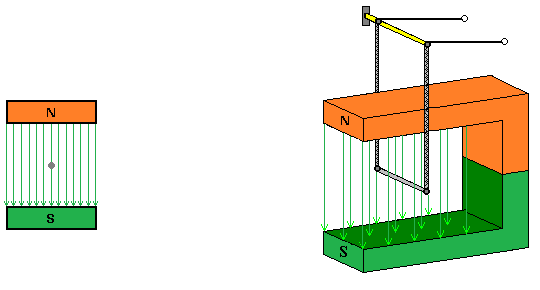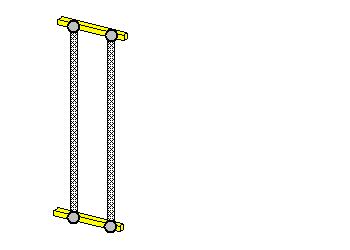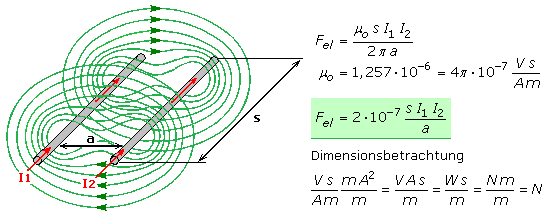# How the current flows through the conductor

## Electromagnetism

The natural scientist Hans Christian Ørsted observed in experiments that a conductor through which an electric current flows deflects a magnetic needle. The current flow in the metallic conductor wire is based on freely moving electrons as charge carriers. When a voltage is applied, they produce the electrical current in a directional movement and generate a magnetic field around the conductor. A few years later, A. M. Ampère constructed an electromagnetic coil and postulated in 1825 that the source of the magnetic forces was to be found in the electric current.

The magnetic field of a straight conductor through which a current flows

The magnetic needles arranged around a vertical conductor rod are aligned with the earth's magnetic field as long as no current flows through the conductor. As soon as electrical current flows, they reorient themselves, whereby their direction of deflection depends on the direction of the current. The electric current builds up a magnetic field around the conductor. The magnetic needles point in the direction that results from the additive superposition of the earth's magnetic field and the conductor magnetic field.

Switch off, switch on, reverse the polarity of the currentWhen the polarity of the current is reversed, the needles orient themselves in the opposite direction. If the current is strong enough, the influence of the earth's magnetic field in the vicinity of the conductor can be neglected. If the needles are on a rotatable plate and the conductor goes through their center point, it can be shown that the magnetic field spreads concentrically around the conductor. The alignment of the magnetic needles is tangential to the electromagnetic field lines.

In another experiment, the conductor rod through which the current flows is supposed to be aligned horizontally in the direction of the earth's magnetic field. Magnetic needles standing parallel on both sides at the same height of the conductor do not experience any deflection. Magnetic needles above and below the conductor are deflected in mutually opposite directions. Both experiments show that the field lines around the conductor form closed circles.

The electromagnetic field of a conductor has no pronounced north or south pole, as is known from bar magnets. With every permanent magnet, the field lines do not end at the poles, but continue in the magnet, so that each field line is self-contained. Magnetic fields are vortex fields without a source or sink. The direction of rotation of the magnetic field depends on the direction of the current. If the technical direction of current from plus to minus is taken as a basis, the direction of rotation can be determined with the right hand. The thumb of the right hand points in the direction of the current and the fingers surrounding the conductor indicate the direction of rotation. The same result is achieved with the so-called corkscrew rule, if it is mentally turned into the conductor in the plus-minus direction of the current. The direction of rotation is identical to the clockwise direction. If the physical direction of the current is from minus to plus, then the thumb of the left hand indicates the direction of the current and the fingers surrounding the conductor show the direction of rotation of the magnetic field. In this case, the direction of rotation of the magnetic field is counterclockwise.

Some time later it was recognized that every moving charge, including electron beams in a vacuum, such as those found in electron beam and gas discharge tubes, generate a magnetic field around them. This gave rise to the possibility of explaining the existence of molecular dipoles in matter. The electrons of the atoms and molecules represent moving charges and generate proportionally very small currents, which in turn build up magnetic fields. The sum of all uncompensated magnetic fields of a material determines its outwardly effective para-, dia- or ferromagnetic properties.

Every electrical current in the form of moving charges creates a magnetic field.
The higher the current, the stronger the magnetic field.
The magnetic field lines form concentric closed circles around the conductor.
The magnetic field plane is perpendicular to the current flow.
Looking in the technical direction of current from plus to minus, the direction of rotation of the magnetic field is directed to the right.

Effect of force on a conductor through which a current flows

If a conductor through which the current flows is located in an external magnetic field, then its magnetic field is superimposed on it. Forces act between the magnetic fields. A conductor of length l is traversed by the current I and is located in an external homogeneous magnetic field. The direction of the current is perpendicular to the external direction of the magnetic field. Both magnetic fields are superimposed, whereby the field is weakened on one side and strengthened on the other. Every field strives to take on its smallest energy content, so the field lines want to shorten. A force F acts on the conductor and deflects the conductor to the weaker side of the field. This force is directly dependent on the permanent magnetic field, the conductor current flow and the conductor length running in the magnetic field. The following interactive picture illustrates these statements.

Turn off the power Turn on the power### Force effect between current-carrying conductors

Another experiment shows the existence and the force effect of the magnetic field. Two metal straps are loosely suspended parallel to each other on insulated clamps. If electrical current flows through both conductors in parallel in the same direction, the ligaments attract each other. If the two conductors are connected in series, the current flows through the ribbons in opposite directions and they repel each other.

Switch off current in the same direction current in the opposite directionIn the first case, the magnetic field lines between the ligaments want to shorten. This causes the attraction as between dissimilar magnetic poles. In the second experiment, the magnetic field lines between the bands run in the same direction. This corresponds to a parallel position of poles of the same name that repel each other.

The magnetic fields of both conductors overlap. A force acts on each conductor that is proportional to the conductor length l and the current intensity I. The force is inversely proportional to the conductor spacing a. The further one moves away from the source of the magnetic field, the weaker the field effect becomes.The equation for calculating the force contains the value μo in the constant of proportionality. It is the magnetic field constant of the vacuum, the value of which also applies with great accuracy to the air-filled room. The circumference with 2 · π · a corresponds to the mean field line length. As a scalar value, the magnitude of the force is independent of the direction of the current. With currents flowing in the same direction, the result is positive, there is attraction. For currents in opposite directions the result is negative, the conductors repel each other.

The force on a section of a 1 m long conductor pair with a distance of 1 m from each other is calculated as 2 · 10exp − 7 N. This corresponded to the old definition for the current strength 1 A.

In the case of very large currents, the force effect of conductor systems running close together should not be neglected. In lead-acid batteries, each positive plate lies between two negative plates. In this way, the repulsive forces in the anode can be canceled out and it is not mechanically stressed. Without this precaution, the injected lead dioxide would loosen and fall out. The result is an internal short circuit between the plates that destroys the battery.